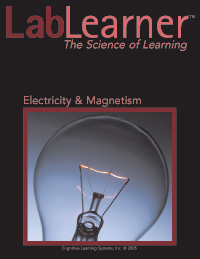# Electricity & MagnetismElectricity & Magnetism

Experiments are centered on the investigation of questions pertaining to the relationships between voltage, current, resistance and magnetism.

Students find that voltage and current are proportionally related, and that the ratio of voltage to current is resistance. They also discover that this relationship between voltage, current, and resistance is defined as Ohm’s law. Students find that increasing voltage increases the amount of current in a circuit while resistance remains constant, regardless of the size of resistor.

Students discover that Ohm’s law is also true when the voltage is held constant while the amount of resistance changes. Increasing the number of resistors has the same effect as changing the diameter or the length of a resistor. Students discover that increasing the length of a resistor will increase the amount of resistance in the circuit. In contrast, increasing the diameter of a resistor increases its cross-sectional area and thus decreases the amount of resistance.

Thirdly, students investigate the role of current in a magnetic field. The magnitude of an electromagnet’s magnetic field is directly related to the number of coils of wire around the magnet, the length of the coil, and the amount of current flowing through the wire. Students discover that increasing current causes an increase in the magnetic field, allowing the magnet to attract a greater number of paper clips. Students also learn through experimentation that increasing the number of coils of wire while keeping the length of the coil constant increases the magnetic field as well.

Finally, students have an opportunity during the performance assessment to put the three concepts together as they create three electromagnets to meet certain specifications. Students are asked to apply their understanding of the fundamentals of Ohm’s law as well as the relationship of current to magnetic field to increase and decrease magnetic field.

Investigation focus:

• Discover that voltage is equal to the amount of current in a system multiplied by the amount of resistance provided by components in the system.
• Learn that Ohm’s law states that the change in current is proportional to the change in voltage in a circuit and that the degree of change is a constant called resistance.
• Learn to represent the relationship between voltage, current and resistance mathematically as R = V/I, where V = voltage, I = current, and R = resistance.
• Discover that there is a direct relationship between voltage and current.
• Discover that the amount of resistance of an electrical component is determined by its length and its cross-sectional area.
• Discover that placing resistors in series results in an increase in resistance due to an increase in the length of the resistor.
• Discover that placing resistors in parallel results in a decrease in resistance due to an increase in the cross-sectional area of the resistor.
• Learn to use a multimeter to determine the voltage and current in a circuit.
• Discover that magnetic field is directly related to the current flowing through an electromagnet.
• Discover that magnetic field is directly related to the number of turns of wire per unit length of an electromagnet.
• Learn to express voltage, current and resistance in proper units of measurement.